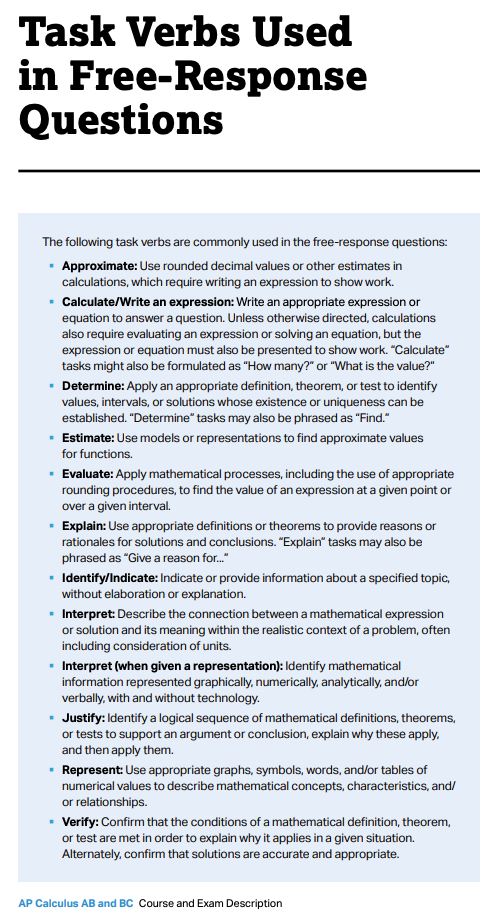# Qtr 3 Study Guide - AP Calculus AB

The exam will have some multiple choice questions, and some response questions, very much how we have Chapter Tests on TestPortals.com. The exam covers all the material we covered from Chapters P to Chapter 7. The review packets are at hw.mathorama.com and the solutions are on plus portal. The material in the review packet includes multiple choice questions from the chapters on the exam. The review packets will refresh your memory of all the concepts covered and will help you practice working on multiple choice type questions. Additional practice can be found on DeltaMath.com, and myAp.collegeboard.com.

The questions on the exam do not require difficult calculations, but an understanding of the concepts. There is more thought than brute force necessary to come up with the correct solutions.
The actual AP Calculus Exams will be one of three dates:

1. (Paper, in school) Tuesday May 4, 2021 8:00 AM PDT
2. (Paper, in school) Monday May 24, 2021 8:00 AM PDT
3. (Digital) Wednesday June 9, 2021 9:00 AM PDT

The College Board (that administers the AP exams) has this helpful passage about what should be included in a free response question:

Students are expected to show enough of their work for Readers to follow their line of reasoning. To obtain full credit for the solution to a free-response problem, students must communicate their methods and conclusions clearly. Answers should show enough work so that the reasoning process can be followed throughout the solution. This is particularly important for assessing partial credit. Students may also be asked to use complete sentences to explain their methods or the reasonableness of their answers, or to interpret their results.

For results obtained using the calculator capabilities of plotting, finding zeros, finding the numerical derivative or integral, students are required to write the setup (e.g., the equation being solved, or the derivative or definite integral being evaluated) that leads to the solution, along with the result produced by the calculator.

For example, if the student is asked to find the area of a region, the student is expected to show a definite integral (i.e., the setup) and the answer. The student need not compute the antiderivative; the calculator may be used to calculate the value of the definite integral without further explanation.

For solutions obtained using the calculator capabilities, students must also show the mathematical steps that lead to the answer; a calculator result is not sufficient. For example, if the student is asked to find a relative minimum value of a function, the student is expected to use calculus and show the mathematical steps that lead to the answer. It is not sufficient to graph the function or use a built-in minimum folder.

When a student is asked to justify an answer, the justification must include mathematical reasons, not merely calculator results. Functions, graphs, tables, or other objects that are used in a justification should be clearly identified.

Our Qtr 3 Exam is only 90 minutes, but will include all ten units of the AP Calculus Course and Exam Description (CED):Here is a check list of the topics:

Here is a check list of the topics:

• Limits
• The definition of continuity
• The Intermediate Value Theorem (IVT)
• The definition of differentiability
• The limit process for finding a derivative
• Differentiating using the power
• Product, quotient, and chain rules
• Implicit differentiation
• Related rates
• Extreme Value Thm (EVT)
• Candidates Test for extrema on a closed interval
• Rolle's Thm, Mean Value Theorem (MVT) for derivatives
• Mean Value Thm (MVT) for integrals
• 1st Derivative and 2nd Derivative Test for extrema
• Curve sketching, limits at infinity
• Optimization Problems
• Antiderivatives and Indefinite Integrals
• Riemann Sums and Definite Integrals
• The Fundamental Theorem of Calculus
• Integration by Substitution (u-sub)
• Slope if inverse functions
• Integration and Differentiation of Exponential and Logarithmic functions
• Indeterminate Forms and L'Hôpital's Rule
• Slope fields
• Recognizing when an equation is the solution of a Differential Equation
• Solving Separable Differential Equations
• Area between 2 functions
• Volume of functions rotated around axes and lines parallel to the axes
• Volume of solids that have a base area that is the area between 2 functions

## Helpful Links

1. Watch videos at passAPcalc.com
2. DeltaMath Practice
3. Khan Academy's AP Calculus AB Web site Has a lot of great interactive assignments that provide hints, solutions, and links to videos that explain every topic on the AP Exam. You would want to go over the assignments for the topics listed above and consult our class at KhanAcademy
4. Khan Academy's AP Calculus AB
5. myAP.collegeboard.org AP Classroom now has short Daily Videos for Units 1-6
6. Past Exam Questions from the College Board.
7. Past Exam Answers from Mr Calculus.
8. Past Exam Answers from Skylit.com.
9. Exam Information from the College Board
10. AP Exam Info
11. Example Multiple choice and Free response questions are in the AP Course Description (Exam questions begin around page 228)
12. MC questions from 1969-1998
13. 2008 Multiple choice Questions and answers
14. Video Links from the homework page
15. Videos from the Mathorama Podcast
16. Worksheets from the class Google classroom page.
17. Syllabus has a grade calculator.

It would be good to go over the Free Response and Multiple choice questions that we started working after the Chapter 7 Test (MC1, FR1, Der-Int-Cal-Thm-Gpg WS for example), the PPC's at myAp.collegeBoard.org, and the AP Calc AB at Khan Academy (In particular the solved exams section), homework and tests; review what you did well, and learn from any mistakes. Another worksheet that might be helpful is a strategy review: AP Calculus:When you see the words....

. The exam category is worth 20% of your grade, and will be curved. (There is a grade calculator in the Syllabus)

Remember to a good night's rest, and eat a healthy breakfast!

Good Luck!